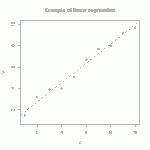# Tutorial: multi-linear regressionThe straight line is the linear regression of a function that takes scalars (x-values) as input and returns scalars (y-values) as output. (figure from GANFYD)

You’ve probably seen classical equations for linear regression, which is a procedure that finds the straight line that best fits a set of discrete points$\{(x_1,y_1), (x_2,y_2),...,(x_N,y_N)\}$. You might also be aware that similar formulas exist to find a straight line that is a best (least squares) fit to a continuous function$y(x)$.The pink parallelogram is the multi-linear regression of a function that takes vectors (gray dots) as input and returns vectors (blue dots) as output

The bottom of this post provides a link to a tutorial on how to generalize the concept of linear regression to fit a function$\vec{y}(\vec{x})$ that takes a vector$\vec{x}$ as input and produces a vector$\vec{y}$ as output. In mechanics, the most common example of this type of function is a mapping function that describes material deformation: the input vector is the initial location of a point on a body, and the output vector is the deformed location of the same point. The image shows a collection of input vectors (initial positions, as grey dots) and a collection of output vectors (deformed locations as blue dots). The affine fit to these descrete data is the pink parallelogram.

Analogous to the scalar formula for a straight line,$y=mx+b$, a mapping transformation is “affine” or “homogeneous” if it is expressible in the form$y_i=M_{ij}x_j+b_i$ (here, we are using Einstein’s implied summation index notation). For an affine mapping, a physical domain that is initially rectangular will deform to a parallelogram. More broadly, any initially straight line of points will deform, under homogeneous mapping, to a new straight line that is possibly of different length and orientation. Otherwise, if any initially straight line becomes curved, then the mapping is nonlinear.

The generalization of linear regression, called multi-linear regression, aims to find the affine mapping that is as close as possible (by minimizing a square mean residual) to a nonlinear mapping. This might be used, for example, in heirarchical multiscale modeling where you seek the overall uniform deformation field that most closely approximates non-uniform deformations in an explicit model of microscale grains. You might also apply multilinear regression to turn a discrete displacement field (such as motion of atoms in a Molecular Dynamics simulation) into a continuum representation of the same motion.

The quality of the fit is visualized by showing the curvy deformed shape of an initially rectangular domain in comparison to the parallelogram deformed shape of the affine fit. [WARNING: deformation of a rectangular boundary to become a parallelogram is necessary, but not sufficient, for a mapping to be affine]. A better visualization would additioinally color the domain by the local residual, as defined in the full write-up linked below.

For the full equations, refer to our tutorial on affine (multilinear) regression. That document refers to some sample data, which are available here as a zip archive